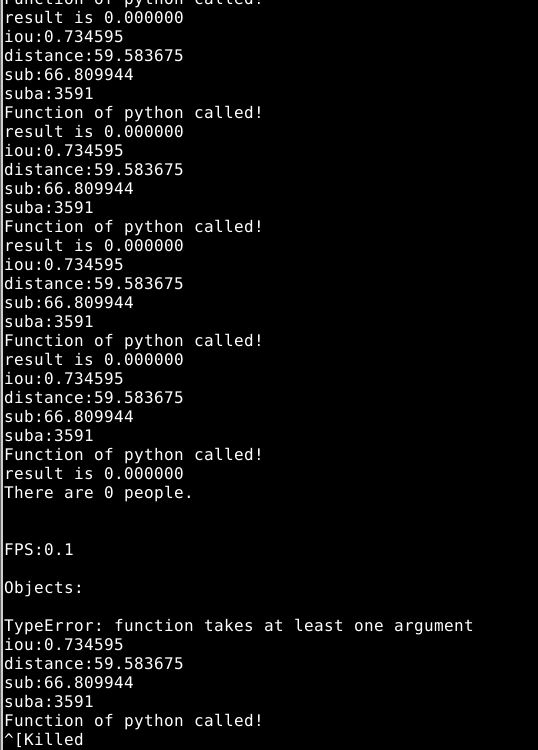srand((unsigned)time(NULL));

``````for (i=0;i<50;i++){

io = rand()/(double)(RAND_MAX);
printf("iou:%f\n",io);

dist = rand()/(double)(RAND_MAX/100);
printf("distance:%f\n",dist);

su = rand()/(double)(RAND_MAX/100);
printf("sub:%f\n",su);

sua = rand()%50000;
printf("suba:%d\n",sua);
Py_Initialize();
if(!Py_IsInitialized())
{
printf("Python init failed!\n");
return;
}
PyRun_SimpleString("import sys");
PyRun_SimpleString("sys.path.append('//home//wangxiangsheng//wuhao//test')");

PyObject *pName = NULL;
PyObject *pModule = NULL;
PyObject *pDict = NULL;
PyObject *pFunc = NULL;
PyObject *pArgs = NULL;
PyObject *pResult = NULL;
pName = PyString_FromString("RF");
pModule = PyImport_Import(pName);
pDict = PyModule_GetDict(pModule);
pFunc = PyDict_GetItemString(pDict,"randomF");

pArgs = Py_BuildValue("(f,f,f,i)",io,dist,su,sua);

pResult = PyObject_CallObject(pFunc,pArgs);
PyArg_Parse(pResult, "i", &res);
printf("result is %d\n",res);
``````
`````` 调用的python脚本：RF.py

``````

import sklearn as skl
from sklearn.externals import joblib
from sklearn.ensemble import RandomForestClassifier

def randomF(iou,distance,sub,suba):

``````test_data = [[iou,distance,sub,suba]]

output = clf.predict(test_data)
print output
return output
````````````     Py_Initialize();
if(!Py_IsInitialized())
{
printf("Python init failed!\n");
return;
}
PyRun_SimpleString("import sys");
PyRun_SimpleString("sys.path.append('//home//wangxiangsheng//darknet//src')");

PyObject *pName = NULL;
PyObject *pModule = NULL;
PyObject *pDict = NULL;
PyObject *pFunc = NULL;
PyObject *pArgs = NULL;
PyObject *pResult = NULL;

pName = PyString_FromString("RF");
pModule = PyImport_Import(pName);
if(!pModule)
{
getchar();
}
pDict = PyModule_GetDict(pModule);
if(!pDict)
{
printf("Can't find dict in RF!\n");
}

pFunc = PyDict_GetItemString(pDict,"randomF");
if(!pFunc || !PyCallable_Check(pFunc))
{
printf("Can't find function!\n");
getchar();
}

for(j=0;j<10;j++){
for(w=j+1;w<39;w++){
float media = box_iou(para[j].p, para[w].p);
float iout;
if (media<=0){
iout = 0.000000;
} else {
iout = media;
}
int p,suba;
float res;
suba = abs(para[j].barea-para[w].barea);
float sub=0;
for(p=0;p<64;p++){
sub = sub + pow(para[j].hist[p]-para[w].hist[p],2);
}
float distance;
distance = sqrt(pow(para[w].p.x-para[j].p.x,2)+pow(para[w].p.y-para[j].p.y,2));
printf("%f-%f-%f-%d \n",iout,distance,sub,suba);
pArgs = Py_BuildValue("(f,f,f,i)",iout,distance,sub,suba);
pResult = PyObject_CallObject(pFunc,pArgs);
PyArg_Parse(pResult, "f", &res);

printf("result is %f\n",res);
``````

1个回答TLedTS emmm。。。我就是参考了这些网站改的，只调用一次的话没有问题，但是重复调用就不能重复Py_Finalize，扔掉这个之后反倒能正常运行。我试验了一个小demo能正常运行我写的程序，就是在嵌入yolo源码中时除了问题。
2 年多之前 回复C语言调用python3出现问题

1. 可以使用python提供C的API进行如下操作吗？ ``` PyRun_SimpleString("import struct") PyRun_SimpleString("import ctypes") ``` 目前测试中发现会出现很多问题，报PyFloat_Type类型undefined；是否需要import其他的东西？ 2. python脚本中增加或者import的其他脚本中增加"import struct"或者"import ctypes"导致C语言无法获取python模块？

C语言代码 #include <stdio.h> #include"Python.h" //这里要包含头文件 //C/C++中执行python文件 void exec_python_file() { //初始化 Py_Initialize(); //choose1，执行单纯的内嵌字符串python代码，建议使用 if(!PyRun_SimpleString("execfile('draw1.py')")) printf("execute python file program failed\n"); //choose2，执行python文件,不建议使用 char fileStr[]="draw1.py"; FILE *fp; if(!(fp=fopen(fileStr,"r"))) printf("open python file failed!\n"); if(!PyRun_SimpleFile(fp,fileStr)) printf("execute python file failed!\n"); fclose(fp); //释放资源 Py_Finalize(); } int main() { exec_python_file(); return 0; } ``` python 代码 #! /usr/bin/python python2.7 # -*- coding:utf-8 -*- import numpy as np import pylab as p1 x1 = [-25,-24,-23,-22,-21,-20,-19,-18,-17,-16,-15,-14,-13,-12,-11,-10,-9,-8,-7,-6,-5,-4,-3,-2,-1,0,1,2,3,4,5,6,7,8,9,10,11,12,13,14,15,16,17,18,19,20,21,22,23,24,25] y1 = [20,25,38,51,103,101,95,90,102,101,235,200,181,110,132,192,381,311,273,145,404,1023,1186,1835,1875,1881,1870,1820,1190,1010,390,150,210,309,379,188,120,108,170,140,230,180,104,94,97,40,38,27,25,22,20] p1.plot(x1,y1,'r') p1.title('Diffraction intensity distribution') p1.xlabel('coord/cm') p1.ylabel('light intensity/mA') p1.xlim(-25.0,25.0) p1.ylim(0.0,1900.0) p1.show() ``` 想实现用C语言调用python 编译时报错: gcc test.c -o test -l/usr/include/python2.7/ -lpyhton2.7 ``` 求解决

【python-C相互调用】python里的dict如何作为参数传入.so中的c语言函数

【python-C相互调用】关于python通过.so调用C函数来读取python里某个key的值并进行处理，一直无法将dict传到C中，求助 1. 首先是C中的包装函数定义 ``` static PyObject * Extest_reverse(PyObject *self, PyObject *args,PyObject *keyds) { //PyObject* keyds = PyDict_Keys(args); static char* kwlist[] = {"name",NULL}; char *name; //将dict中的name里的值读到char* name中 PyObject* retval; if(PyArg_ParseTupleAndKeywords(args,keyds,"isi",kwlist,&name)) { retval = (PyObject *)Py_BuildValue("i",1); printf("%s",name); free(name); return retval; //成功读取返回1 } else retval = (PyObject *)Py_BuildValue("i",0); return retval; //未读取返回0 } static PyMethodDef ExtestMethods[] = { {"reverse",(PyCFunction)Extest_reverse,METH_VARARGS | METH_KEYWORDS,"reverse"}, }; void initwmf() { Py_InitModule("wmf",ExtestMethods); } ``` 2. python里的调用 .so已生成，将python 中定义的dict作为参数输入 ``` import wmf test = {'name':'wmf'} result = wmf.reverse(test) print(result) ``` 返回结果一直是0，未读到对应key值的value，进一步查验返现，test这个dict就没有传进去，传入参数是NULL。求助定义的问题，还是调用的问题？如何解决

C语言代码如何转换为python代码？linux和windows的操作分别是什么？

nodejs 调用c语言程序传参问题

var strCmd = ./test.elf -e title exec(strCmd, g_strExecOptions, function(err, stdout, stderr) {}）; test.elf为c语言程序，title为汉字字符串，C语言的命令行参数解析使用glib的g_option_context_parse函数，但是，每次调用的时候都提示 option parsing failed: Invalid byte sequence in conversion input/n，请教以下高手，告知原因。nodejs采用的是utf8编码。

c语言，程序中函数没被调用。

```#include<stdio.h> #include<malloc.h> #include<string.h> #include<stdlib.h> #define OK 0 #define Err_Memory -1 #define Err_InvalidParam -2 #define Err_Overflow -3 #define Err_IllegalPos -4 #define Err_NoResult -5 typedef char *ElemType ; typedef struct node{ ElemType data; struct node *next; }ListNode,*LinkList; typedef int Status; Status InitList (LinkList L) { if (!L) return Err_InvalidParam ; L->next=NULL; return OK; } Status CreateCollection (LinkList C) { int count=1; ListNode *s,*p; ElemType e; if (InitList!=OK) return Err_NoResult ; p=C; while (1) { s=(ListNode *)malloc(sizeof(ListNode)); if (!s) return Err_Memory; e=(ElemType)malloc(11*sizeof(char)); if (!e) return Err_Memory; printf("请输入第%d个元素:",count++); gets(e); if (strcmp(e,"#")==0) break ; if (LocataList(e,C)==0) { if (InsertList(p,1,e)!=OK) return Err_NoResult ; p=p->next; } } return OK; } int LocateList (ElemType e,LinkList L) { int i=1; ListNode *p; if (!L) return Err_InvalidParam ; p=L->next; while (p&&strcmp (p->data,e)!=0) { i++; p=p->next; } if (!p) return 0; return i; } Status InsertList (LinkList L,int i,ElemType e) { ListNode *p,*s; int k=1; if (!L) return Err_InvalidParam ; p=L; while (k<i&&p) { k++; p=p->next; } if (k>i||!p) return Err_IllegalPos; s=(ListNode *)malloc(sizeof(ListNode)); if (!s) return Err_Memory; s->data=e; s->next=p->next; p->next=s; return OK; } int main() { LinkList A,B,C; ElemType s; A=(ListNode *)malloc(sizeof(ListNode)); B=(ListNode *)malloc(sizeof(ListNode)); C=(ListNode *)malloc(sizeof(ListNode)); printf("请输入集合A的元素（输入#结束）：\n"); //scanf("%s",A); if (CreateCollection(A)!=OK) { printf("集合A创建错误！"); getch();return ;} ``` ![图片说明](https://img-ask.csdn.net/upload/201609/12/1473647353_427897.png) 请问一下，为什么这里，CreateCollection 函数没有被调用呢？ 初学数据结构。 补贴了Insert 和Locate 函数。

C++调用Python加载第三方库速度很慢怎么解决？

c与python互相调用传递参数

C调用Python怎么向python里传递结构体，怎么将一个python模块添加到python的一个函数里面，

C语言中函数数组调用问题

c语言函数嵌套调用疑惑

/* 显示unsigned型的位串 */ #include <stdio.h> // 返回在整数x中设置的位数 int count_bits(unsigned x) { int count = 0; while (x) { if (x & 1U) count++; x >>= 1; } return (count); } // 返回unsigned型的位数 int int_bits(void) { return (count_bits(~0U)); } // 显示unsigned型的位串内容 void print_bits(unsigned x) { int i; for (i = int_bits() - 1; i >= 0; i--) putchar(((x >> i) & 1U) ? '1' : '0'); } int main(void) { unsigned nx; printf("请输入一个非负整数："); scanf("%u", &nx); print_bits(nx); putchar('\n'); return (0); } 在自学c语言，有一个小程序看不懂了，来请教大家main函数调用print_bits函数，print_bits函数在调用int_bits函数到这一步就看不懂了大家能给仔细 讲讲这个函数？

c语言关于主函数调用子函数实现特定功能

C语言中函数调用出错问题的解决方法，主函数中的函数参数问题

C语言中函数调用参数的问题......

for (ctr = 0; ctr <= numMov; ctr++) { printf("请输入电影的名字\n\n"); scanf_s("%c", namMov); printf("请对该电影进行评分！\n\n"); scanf("%d", &rating); if (rating>fisRating) { strcpy_s(favorite, namMov); -- 程序在此指出错误：函数调用中的参数太少 fisRating = rating; 请问应该怎么解决？？？

c语言程序调用了动态链接库，如果想将最后的c程序再做成动态链接库，可行么？

C语言中main调用一个接口函数问题。

c语言函数调用中的调用参数

⑴ 编写一个汇编语言递归子程序（子模块）求斐波那契数列，斐波纳契数列以如下被以递归的方法定义：F(0)=0，F(1)=1，F(n)=F(n-1)+F(n-2)（n>=2，n∈N*）；（输入一个数字，若该数字在数列中，则输出N,若不在数列中，输出“error”） ⑵ 编写一个计算Z=sin(2n+1)*F(n)的汇编语言主模块，其中sin(x)调用C语言的函数库、F(n)通过调用⑴中的模块实现，输入n。显示格式为： Z= 使用调试程序观察参数传递及堆栈平衡情况。

linux下利用/proc进行进程树的打印

Python数据分析与挖掘

92讲视频课+16大项目实战+源码+￥800元课程礼包+讲师社群1V1答疑+社群闭门分享会=99元 &nbsp; 为什么学习数据分析？ &nbsp; &nbsp; &nbsp; 人工智能、大数据时代有什么技能是可以运用在各种行业的？数据分析就是。 &nbsp; &nbsp; &nbsp; 从海量数据中获得别人看不见的信息，创业者可以通过数据分析来优化产品，营销人员可以通过数据分析改进营销策略，产品经理可以通过数据分析洞察用户习惯，金融从业者可以通过数据分析规避投资风险，程序员可以通过数据分析进一步挖掘出数据价值，它和编程一样，本质上也是一个工具，通过数据来对现实事物进行分析和识别的能力。不管你从事什么行业，掌握了数据分析能力，往往在其岗位上更有竞争力。 &nbsp;&nbsp; 本课程共包含五大模块： 一、先导篇： 通过分析数据分析师的一天，让学员了解全面了解成为一个数据分析师的所有必修功法，对数据分析师不在迷惑。 &nbsp; 二、基础篇： 围绕Python基础语法介绍、数据预处理、数据可视化以及数据分析与挖掘......这些核心技能模块展开，帮助你快速而全面的掌握和了解成为一个数据分析师的所有必修功法。 &nbsp; 三、数据采集篇： 通过网络爬虫实战解决数据分析的必经之路：数据从何来的问题，讲解常见的爬虫套路并利用三大实战帮助学员扎实数据采集能力，避免没有数据可分析的尴尬。 &nbsp; 四、分析工具篇： 讲解数据分析避不开的科学计算库Numpy、数据分析工具Pandas及常见可视化工具Matplotlib。 &nbsp; 五、算法篇： 算法是数据分析的精华，课程精选10大算法，包括分类、聚类、预测3大类型，每个算法都从原理和案例两个角度学习，让你不仅能用起来，了解原理，还能知道为什么这么做。

Only老K说-爬取妹子图片（简单入门）

linux“开发工具三剑客”速成攻略

Python代码实现飞机大战

Python数据清洗实战入门

2019 Python开发者日-培训

apache-jmeter-5.1.1(Requires Java 8+).zip

。Apache JMeter 5.1.1 (Requires Java 8+)，需要jdk8以上的版本。

Python可以这样学（第四季：数据分析与科学计算可视化）

Java基础知识面试题（2020最新版）

2019 AI开发者大会

2019 AI开发者大会(AI ProCon 2019)是由中国IT社区CSDN主办的AI技术与产业年度盛会。多年经验淬炼，如今蓄势待发：2019年9月6-7日，大会将有近百位中美顶尖AI专家、知名企业代表以及千余名AI开发者齐聚北京，进行技术解读和产业论证。我们不空谈口号，只谈技术，诚挚邀请AI业内人士一起共铸人工智能新篇章！

Python数据挖掘简易入门

&nbsp; &nbsp; &nbsp; &nbsp; 本课程为Python数据挖掘方向的入门课程，课程主要以真实数据为基础，详细介绍数据挖掘入门的流程和使用Python实现pandas与numpy在数据挖掘方向的运用，并深入学习如何运用scikit-learn调用常用的数据挖掘算法解决数据挖掘问题，为进一步深入学习数据挖掘打下扎实的基础。

C/C++学习指南全套教程

C/C++学习的全套教程，从基本语法，基本原理，到界面开发、网络开发、Linux开发、安全算法，应用尽用。由毕业于清华大学的业内人士执课，为C/C++编程爱好者的教程。

2021考研数学张宇基础30讲.pdf

<p> 限时福利限时福利，<span>15000+程序员的选择！</span> </p> <p> 购课后添加学习助手（微信号：csdn590），按提示消息领取编程大礼包！并获取讲师答疑服务！ </p> <p> <br> </p> <p> 套餐中一共包含5门程序员必学的数学课程（共47讲） </p> <p> 课程1：《零基础入门微积分》 </p> <p> 课程2：《数理统计与概率论》 </p> <p> 课程3：《代码学习线性代数》 </p> <p> 课程4：《数据处理的最优化》 </p> <p> 课程5：《马尔可夫随机过程》 </p> <p> <br> </p> <p> 哪些人适合学习这门课程？ </p> <p> 1）大学生，平时只学习了数学理论，并未接触如何应用数学解决编程问题； </p> <p> 2）对算法、数据结构掌握程度薄弱的人，数学可以让你更好的理解算法、数据结构原理及应用； </p> <p> 3）看不懂大牛代码设计思想的人，因为所有的程序设计底层逻辑都是数学； </p> <p> 4）想学习新技术，如：人工智能、机器学习、深度学习等，这门课程是你的必修课程； </p> <p> 5）想修炼更好的编程内功，在遇到问题时可以灵活的应用数学思维解决问题。 </p> <p> <br> </p> <p> 在这门「专为程序员设计的数学课」系列课中，我们保证你能收获到这些:<br> <br> <span> </span> </p> <p class="ql-long-24357476"> <span class="ql-author-24357476">①价值300元编程课程大礼包</span> </p> <p class="ql-long-24357476"> <span class="ql-author-24357476">②应用数学优化代码的实操方法</span> </p> <p class="ql-long-24357476"> <span class="ql-author-24357476">③数学理论在编程实战中的应用</span> </p> <p class="ql-long-24357476"> <span class="ql-author-24357476">④程序员必学的5大数学知识</span> </p> <p class="ql-long-24357476"> <span class="ql-author-24357476">⑤人工智能领域必修数学课</span> </p> <p> <br> 备注：此课程只讲程序员所需要的数学，即使你数学基础薄弱，也能听懂，只需要初中的数学知识就足矣。<br> <br> 如何听课？ </p> <p> 1、登录CSDN学院 APP 在我的课程中进行学习； </p> <p> 2、登录CSDN学院官网。 </p> <p> <br> </p> <p> 购课后如何领取免费赠送的编程大礼包和加入答疑群？ </p> <p> 购课后，添加助教微信：<span> csdn590</span>，按提示领取编程大礼包，或观看付费视频的第一节内容扫码进群答疑交流！ </p> <p> <img src="https://img-bss.csdn.net/201912251155398753.jpg" alt=""> </p>

DDR5_Draft_Spec_Rev05c.pdf

DDR5 spec

Java面试史上最全的JAVA专业术语面试100问 (前1-50)

C/C++跨平台研发从基础到高阶实战系列套餐

Python界面版学生管理系统

2019数学建模A题高压油管的压力控制 省一论文即代码

2019数学建模A题高压油管的压力控制省一完整论文即详细C++和Matlab代码，希望对同学们有所帮助

4小时玩转微信小程序——基础入门与微信支付实战